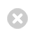Close

MPHY0021: Research Software Engineering With Python

Home## How to Test¶

### Equivalence partitioning¶

Think hard about the different cases the code will run under: this is science, not coding!

We can't write a test for every possible input: this is an infinite amount of work.

We need to write tests to rule out different bugs. There's no need to separately test equivalent inputs.

Let's look at an example of this question outside of coding:

• Research Project : Evolution of agricultural fields in Saskatchewan from aerial photography
• In silico translation : Compute overlap of two rectangles
In :
import matplotlib.pyplot as plt
from matplotlib.path import Path
import matplotlib.patches as patches
%matplotlib inline


Let's make a little fragment of matplotlib code to visualise a pair of fields.

In :
def show_fields(field1, field2):
def vertices(left, bottom, right, top):
verts = [(left, bottom),
(left, top),
(right, top),
(right, bottom),
(left, bottom)]
return verts

codes = [Path.MOVETO,
Path.LINETO,
Path.LINETO,
Path.LINETO,
Path.CLOSEPOLY]
path1 = Path(vertices(*field1), codes)
path2 = Path(vertices(*field2), codes)
fig = plt.figure()
patch1 = patches.PathPatch(path1, facecolor='orange', lw=2)
patch2 = patches.PathPatch(path2, facecolor='blue', lw=2)
ax.set_xlim(0,5)
ax.set_ylim(0,5)

show_fields((1.,1.,4.,4.), (2.,2.,3.,3.))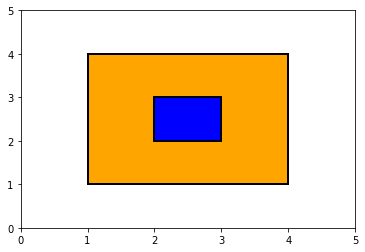Here, we can see that the area of overlap, is the same as the smaller field, with area 1.

We could now go ahead and write a subroutine to calculate that, and also write some test cases for our answer.

But first, let's just consider that question abstractly, what other cases, not equivalent to this might there be?

For example, this case, is still just a full overlap, and is sufficiently equivalent that it's not worth another test:

In :
show_fields((1.,1.,4.,4.),(2.5,1.7,3.2,3.4))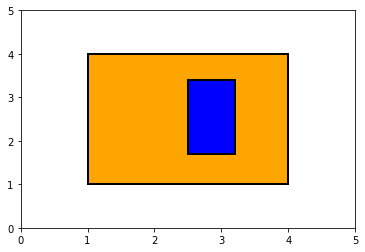But this case is no longer a full overlap, and should be tested separately:

In :
show_fields((1.,1.,4.,4.),(2.,2.,3.,4.5))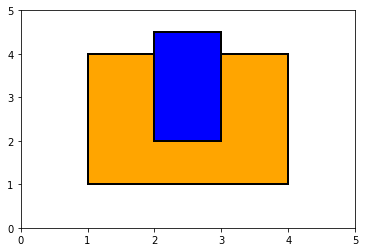On a piece of paper, sketch now the other cases you think should be treated as non-equivalent. Some answers are below:

In :
for _ in range(10):
print("\n\n\nSpoiler space\n\n\n")



Spoiler space

Spoiler space

Spoiler space

Spoiler space

Spoiler space

Spoiler space

Spoiler space

Spoiler space

Spoiler space

Spoiler space


In :
show_fields((1.,1.,4.,4.),(2,2,4.5,4.5)) # Overlap corner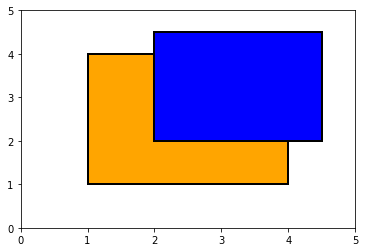In :
show_fields((1.,1.,4.,4.),(2.,2.,3.,4.)) # Just touching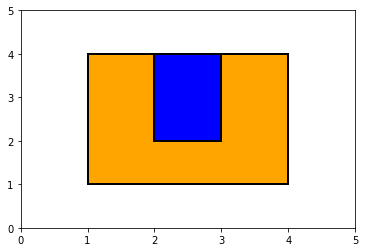In :
show_fields((1.,1.,4.,4.),(4.5,4.5,5,5)) # No overlap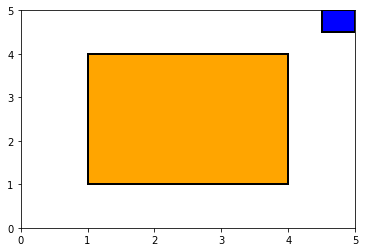In :
show_fields((1.,1.,4.,4.),(2.5,4,3.5,4.5)) # Just touching from outside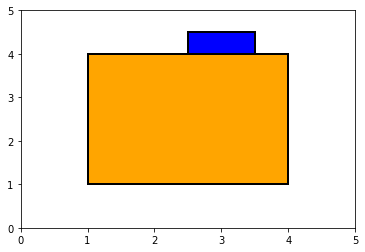In :
show_fields((1.,1.,4.,4.),(4,4,4.5,4.5)) # Touching corner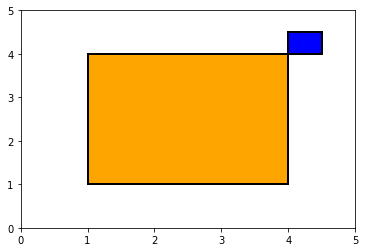### Using our tests¶

OK, so how might our tests be useful?

Here's some code that might correctly calculate the area of overlap:

In :
def overlap(field1, field2):
left1, bottom1, top1, right1 = field1
left2, bottom2, top2, right2 = field2
overlap_left = max(left1, left2)
overlap_bottom = max(bottom1, bottom2)
overlap_right = min(right1, right2)
overlap_top = min(top1, top2)
overlap_height = (overlap_top-overlap_bottom)
overlap_width = (overlap_right-overlap_left)
return overlap_height * overlap_width


So how do we check our code?

The manual approach would be to look at some cases, and, once, run it and check:

In :
overlap((1.,1.,4.,4.),(2.,2.,3.,3.))

Out:
1.0

That looks OK.

But we can do better, we can write code which raises an error if it gets an unexpected answer:

In :
assert overlap((1.,1.,4.,4.),(2.,2.,3.,3.)) == 1.0

In :
assert overlap((1.,1.,4.,4.),(2.,2.,3.,4.5)) == 2.0

In :
assert overlap((1.,1.,4.,4.),(2.,2.,4.5,4.5)) == 4.0

In :
assert overlap((1.,1.,4.,4.),(4.5,4.5,5,5)) == 0.0

---------------------------------------------------------------------------
AssertionError                            Traceback (most recent call last)
/tmp/ipykernel_13602/2498878511.py in <module>
----> 1 assert overlap((1.,1.,4.,4.),(4.5,4.5,5,5)) == 0.0

AssertionError: 
In :
print(overlap((1.,1.,4.,4.),(4.5,4.5,5,5)))

0.25

In :
show_fields((1.,1.,4.,4.),(4.5,4.5,5,5))What? Why is this wrong?

In our calculation, we are actually getting:

In :
overlap_left = 4.5
overlap_right = 4
overlap_width = -0.5
overlap_height = -0.5


Both width and height are negative, resulting in a positive area. The above code didn't take into account the non-overlap correctly.

It should be:

In :
def overlap(field1, field2):
left1, bottom1, top1, right1 = field1
left2, bottom2, top2, right2 = field2

overlap_left = max(left1, left2)
overlap_bottom = max(bottom1, bottom2)
overlap_right = min(right1, right2)
overlap_top = min(top1, top2)

overlap_height = max(0, (overlap_top-overlap_bottom))
overlap_width = max(0, (overlap_right-overlap_left))

return overlap_height*overlap_width

In :
assert overlap((1,1,4,4), (2,2,3,3)) == 1.0
assert overlap((1,1,4,4), (2,2,3,4.5)) == 2.0
assert overlap((1,1,4,4), (2,2,4.5,4.5)) == 4.0
assert overlap((1,1,4,4), (4.5,4.5,5,5)) == 0.0
assert overlap((1,1,4,4), (2.5,4,3.5,4.5)) == 0.0
assert overlap((1,1,4,4), (4,4,4.5,4.5)) == 0.0


Note, we reran our other tests, to check our fix didn't break something else. (We call that "fallout")

### Boundary cases¶

"Boundary cases" are an important area to test:

• Limit between two equivalence classes: edge and corner sharing fields
• Wherever indices appear, check values at 0, N, N+1
• Empty arrays:
atoms = [read_input_atom(input_atom) for input_atom in input_file]
energy = force_field(atoms)

• What happens if atoms is an empty list?
• What happens when a matrix/data-frame reaches one row, or one column?

### Positive and negative tests¶

• Positive tests: code should give correct answer with various inputs
• Negative tests: code should crash as expected given invalid inputs, rather than lying

Bad input should be expected and should fail early and explicitly.

Testing should ensure that explicit failures do indeed happen.

### Raising exceptions¶

In Python, we can signal an error state by raising an error:

In :
def I_only_accept_positive_numbers(number):
# Check input
if number < 0:
raise ValueError("Input {} is negative".format(number))

# Do something

In :
I_only_accept_positive_numbers(5)

In :
I_only_accept_positive_numbers(-5)

---------------------------------------------------------------------------
ValueError                                Traceback (most recent call last)
/tmp/ipykernel_13602/2014601904.py in <module>
----> 1 I_only_accept_positive_numbers(-5)

/tmp/ipykernel_13602/3797577618.py in I_only_accept_positive_numbers(number)
2     # Check input
3     if number < 0:
----> 4         raise ValueError("Input {} is negative".format(number))
5
6     # Do something

ValueError: Input -5 is negative

There are standard "Exception" types, like ValueError we can raise

We would like to be able to write tests like this:

In :
assert I_only_accept_positive_numbers(-5) == # Gives a value error

  File "/tmp/ipykernel_13602/3187101134.py", line 1
assert I_only_accept_positive_numbers(-5) == # Gives a value error
^
SyntaxError: invalid syntax


But to do that, we need to learn about more sophisticated testing tools, called "test frameworks".